# Long Run World Economic Growth

Economics and International Development, LSE
Background Common to Projects
Long-Run World Economic Growth
October 2014
D. Quah

This writeup summarises some useful information on long-run world economic growth using data from the Maddison Project.

``````library(knitr)
opts_chunk\$set(echo=TRUE, tidy=FALSE, warning=FALSE)
setwd("~/Dropbox/1/j/Code/2014.03-World-Growth")``````

The Maddison Project provides the now-standard data to study comparative economic growth over the very long run. These data are provided as an Excel spreadsheet. Unfortunately, that information is given in a way that is more useful visually than for data manipulation and analysis. So, typically, one would need to go through the following to put the data into a more usable form.

``````library(ggplot2)
library(gdata)``````
``````## gdata: read.xls support for 'XLS' (Excel 97-2004) files ENABLED.
##
## gdata: read.xls support for 'XLSX' (Excel 2007+) files ENABLED.
##
## Attaching package: 'gdata'
##
## The following object is masked from 'package:stats':
##
##     nobs
##
## The following object is masked from 'package:utils':
##
##     object.size``````
``````library(reshape2)
library(stringr)``````
``````theMaddisonXLS <- "~/Dropbox/1/j/data/Maddison-Project/mpd_2013-01.xlsx"
colNames       <- as.character(hold.DF[1, ])
colNames    <- "Year"
new.DF         <- hold.DF[-1, ]
names(new.DF)  <- colNames
MaddP.DF       <- melt(new.DF, id.vars="Year")
rm(new.DF, hold.DF, colNames)

``````detach("package:stringr")
detach("package:reshape2")
detach("package:gdata")``````

(You can check that if we didn’t do this trimming of whitespace with`str_trim()`, we wouldn’t get a match for “Sweden” in the codechunk to follow. Astounding but true: Spreadsheets and casual hand-editing are dangerous things to mix and then have lying around on a computer.)

Since I knew I would want to use these data repeatedly and I didn’t want to keep running the codechunk above, I saved my own copy of the Maddison Project GDP data in R’s native format:

``myMaddP.file <- "~/Dropbox/1/j/data/Maddison-Project/maddp-201301-DQ.rds"``
``saveRDS(MaddP.DF, file=myMaddP.file)``

(This is just for my personal use so I’m not packaging it up as a library.)

When I now need to use these data I no longer need to do all the stripping and cleaning after (slowly) reading a spreadsheet as above. Instead I just go:

``MaddP.DF <- readRDS(myMaddP.file)``

and to get growth rates to study subsequently:

``````MaddP.DF\$annGrowth <- NA
for (anEconomy in unique(MaddP.DF\$Economy)) {
theAnnGr <- rep(NA, length(logPCGDP))
for (jLoop in 2:length(theAnnGr)) {
if (theYears[jLoop-1] == theYears[jLoop]-1) {
theAnnGr[jLoop] <- logPCGDP[jLoop] - logPCGDP[jLoop-1]
}
}
# Change to percent and then move into dataframe
rm(theAnnGr)
}``````

(for those who know R, notice I can’t vectorise the inner loop using, say,`diff` as I need to check if the data are available sequentially in time).

What economies are we working with here?

``unique(MaddP.DF\$Economy)``
``````##    "Austria"
##    "Belgium"
##    "Denmark"
##    "Finland"
##    "France"
##    "Germany"
##    "(Centre-   North)           Italy"
##    "Holland/     Netherlands"
##    "Norway"
##   "Sweden"
##   "Switzerland"
##   "England/GB/UK"
##   "12 W. Europe"
##   "Ireland"
##   "Greece"
##   "Portugal"
##   "Spain"
##   "14 small WEC"
##   "30 W. Europe"
##   "Australia"
##   "N. Zealand"
##   "USA"
##   "W. Offshoots"
##   "Albania"
##   "Bulgaria"
##   "Czecho-slovakia"
##   "Hungary"
##   "Poland"
##   "Romania"
##   "Yugoslavia"
##   "7 E. Europe"
##   "Bosnia"
##   "Croatia"
##   "Macedonia"
##   "Slovenia"
##   "Montenegro"
##   "Serbia"
##   "Kosovo"
##   "F. Yugoslavia"
##   "Czech Rep."
##   "Slovakia"
##   "F. Czecho-slovakia"
##   "Armenia"
##   "Azerbaijan"
##   "Belarus"
##   "Estonia"
##   "Georgia"
##   "Kazakhstan"
##   "Kyrgyzstan"
##   "Latvia"
##   "Lithuania"
##   "Moldova"
##   "Russia"
##   "Tajikistan"
##   "Turk-menistan"
##   "Ukraine"
##   "Uzbekistan"
##   "F. USSR"
##   "Argentina"
##   "Brazil"
##   "Chile"
##   "Colombia"
##   "Mexico"
##   "Peru"
##   "Uruguay"
##   "Venezuela"
##   "8 L. America"
##   "Bolivia"
##   "Costa Rica"
##   "Cuba"
##   "Dominican Rep."
##   "El Salvador"
##   "Guatemala"
##   "HaÃ¯ti"
##   "Honduras"
##   "Jamaica"
##   "Nicaragua"
##   "Panama"
##   "Paraguay"
##   "Puerto Rico"
##   "T. &amp; Tobago"
##   "15 L. America"
##   "21 Caribbean"
##   "L. America"
##   "China"
##   "India"
##   "Indonesia (Java before 1880)"
##   "Japan"
##   "Philippines"
##   "S. Korea"
##   "Thailand"
##   "Taiwan"
##   "Burma"
##   "Hong Kong"
##   "Malaysia"
##   "Nepal"
##  "Pakistan"
##  "Singapore"
##  "Sri Lanka"
##  "16 E. Asia"
##  "Afghanistan"
##  "Cambodia"
##  "Laos"
##  "Mongolia"
##  "North Korea"
##  "Vietnam"
##  "24 Sm. E. Asia"
##  "30 E. Asia"
##  "Bahrain"
##  "Iran"
##  "Iraq"
##  "Israel"
##  "Jordan"
##  "Kuwait"
##  "Lebanon"
##  "Oman"
##  "Qatar"
##  "Saudi Arabia"
##  "Syria"
##  "NA"
##  "UAE"
##  "Yemen"
##  "W. Bank &amp; Gaza"
##  "15 W. Asia"
##  "Asia"
##  "Algeria"
##  "Angola"
##  "Benin"
##  "Botswana"
##  "Burkina Faso"
##  "Burundi"
##  "Cameroon"
##  "Cape Verde"
##  "Centr. Afr. Rep."
##  "Comoro Islands"
##  "Congo 'Brazzaville'"
##  "CÃ´te d'Ivoire"
##  "Djibouti"
##  "Egypt"
##  "Equatorial Guinea"
##  "Eritrea &amp; Ethiopia"
##  "Gabon"
##  "Gambia"
##  "Ghana"
##  "Guinea"
##  "Guinea Bissau"
##  "Kenya"
##  "Lesotho"
##  "Liberia"
##  "Libya"
##  "Malawi"
##  "Mali"
##  "Mauritania"
##  "Mauritius"
##  "Morocco"
##  "Mozambique"
##  "Namibia"
##  "Niger"
##  "Nigeria"
##  "Rwanda"
##  "Sao TomÃ© &amp; Principe"
##  "Senegal"
##  "Seychelles"
##  "Sierra Leone"
##  "Somalia"
##  "Cape Colony/ South Africa"
##  "Sudan"
##  "Swaziland"
##  "Tanzania"
##  "Togo"
##  "Tunisia"
##  "Uganda"
##  "Congo-Kinshasa"
##  "Zambia"
##  "Zimbabwe"
##  "3 Small Afr."
##  "Total Africa"
##  "Total World"``````

A bit of a mess, isn’t it? And this is after we’ve already done a `str_trim()`.
Look, I am in awe of the amount of work that has gone into constructing these Maddison Project data. These researchers have my greatest respect. But the mess above is what happens when authors use names they go around making up, like “England/GB/UK”, “Holland/ Netherlands”, “(Centre- North) Italy”, “14 small WEC”, or “3 Small Afr.”; or when they insert peculiar characters like “&” or random invisible whitespace or other non-ASCII characters.

(Without ISO standardisation, of course, it’s inevitable we have to make things up. Still.)

It’s bad enough when we have to guess what these names mean in a spreadsheet; trying to write computer code to select things systematically from this is almost impossible.

But having done our best to clean these data, take a look at some selected growth experiences. For convenience and aesthetics, set up a theme for the charts to come.

``````myTStheme <- theme_classic() +
theme(
plot.title=element_text(size=rel(1.5)),
legend.title=element_text(size=rel(1.5)),
legend.text=element_text(size=rel(1.5)),
legend.position=c(1,0), legend.justification=c(1,0),
axis.text=element_text(size=rel(1.5)),
axis.title=element_text(size=rel(1.5)),
axis.title.y=element_blank()
)``````

In the Appendix I set up R code to do this conveniently; that code will be re-used subsequently as well.

Using that code now, check out these four economies from 1870 to 2010:

``````theBegSmpl   <- 1870
theEndSmpl   <- 2010
theEconomies <- c("USA", "England/GB/UK", "France", "Sweden")
theSeries    <- "logPerCapGDP"
thisTitle    <- "log Per Capita GDP in constant 1990 Int. GK\$"

source(file="./multplot.R", local=TRUE, echo=TRUE)``````
``````##
## > getSeries <- c("Year", "Economy", theSeries)
##
## > theAES <- aes_string(x = "Year", y = theSeries, group = "Economy",
## +     colour = "Economy")
##
## > this.DF <- MaddP.DF[(MaddP.DF\$Economy %in% theEconomies) &
## +     (MaddP.DF\$Year >= theBegSmpl) & (MaddP.DF\$Year <= theEndSmpl),
## +     getSeries]
##
## > ggplot(data = this.DF, theAES) + geom_line(size = 2) +
## +     myTStheme + ggtitle(thisTitle)``````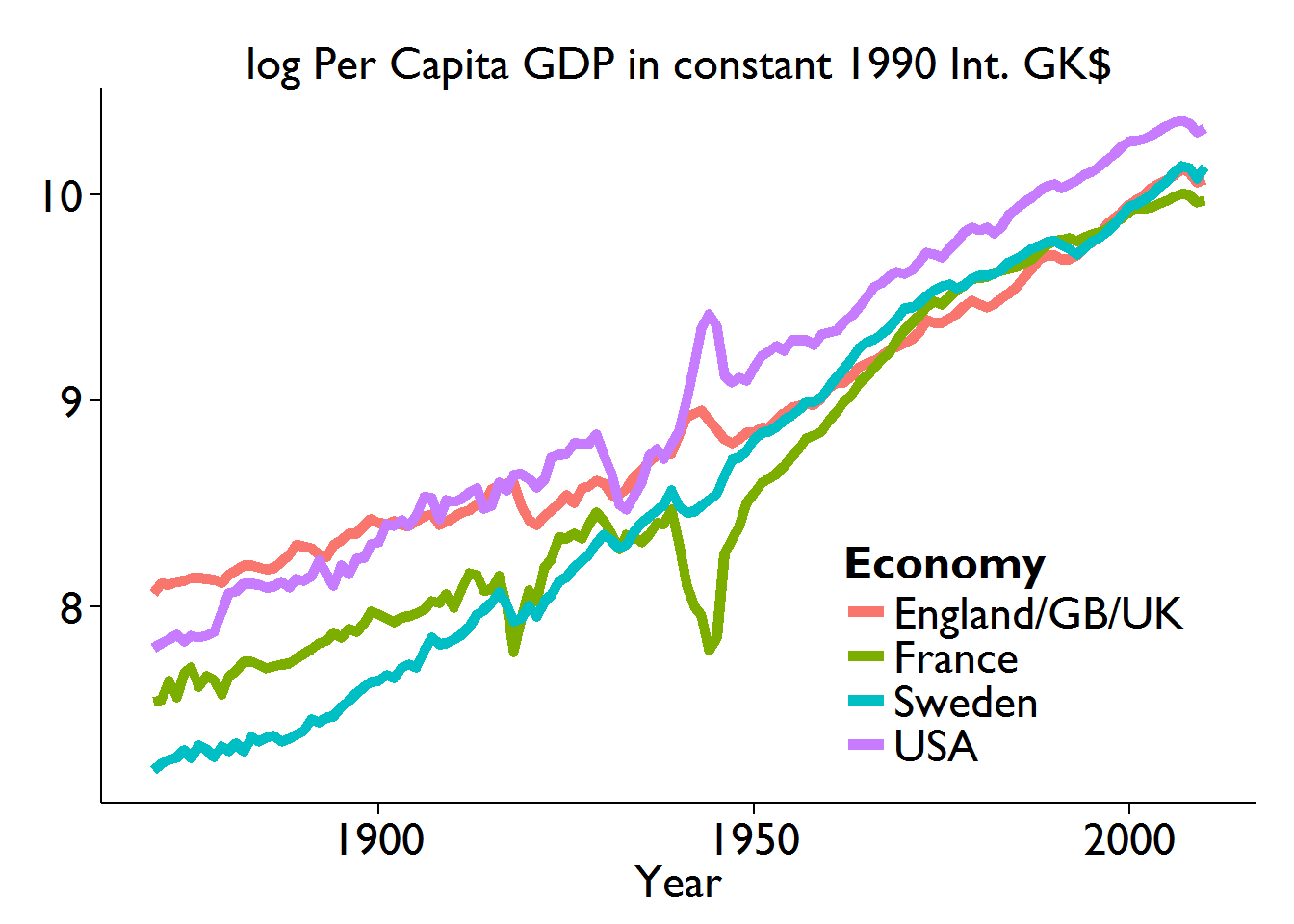``````##
## > rm(this.DF, theAES, getSeries)``````
``rm(thisTitle, theSeries, theEconomies, theEndSmpl, theBegSmpl)``

To structure more clearly this information, I seek to eyeball an extrapolated trend in these per capita incomes data. As previously, I provide in the Appendix the R code to do this. Here, I just call that code after setting up the things I want to see.

Begin with US data:

``````theBegFit  <- 1870
theEndFit  <- 1980
theEndSmp  <- 2010
theEconomy <- "USA"
source(file="./eyetrend.R", local=TRUE, echo=TRUE)``````
``````##
## > olsFIT <- lm(logPerCapGDP ~ Year, data = MaddP.DF[(MaddP.DF\$Economy ==
## +     theEconomy) & (MaddP.DF\$Year >= theBegFit) & (MaddP.DF\$Year <=
## +      .... [TRUNCATED]
##
## > thisTitle <- paste0(theEconomy, ": log Per Capita GDP in constant 1990 Int. GK\$")
##
## > this.DF <- MaddP.DF[(MaddP.DF\$Economy == theEconomy) &
## +     (MaddP.DF\$Year >= theBegFit) & (MaddP.DF\$Year <= theEndSmp),
## +     c("Year", "perCapi ..." ... [TRUNCATED]
##
## > ggplot(data = this.DF, aes(x = Year, y = logPerCapGDP)) +
## +     geom_line(size = 2) + geom_segment(data = this.DF, aes(x = theBegFit,
## +     xend = .... [TRUNCATED]``````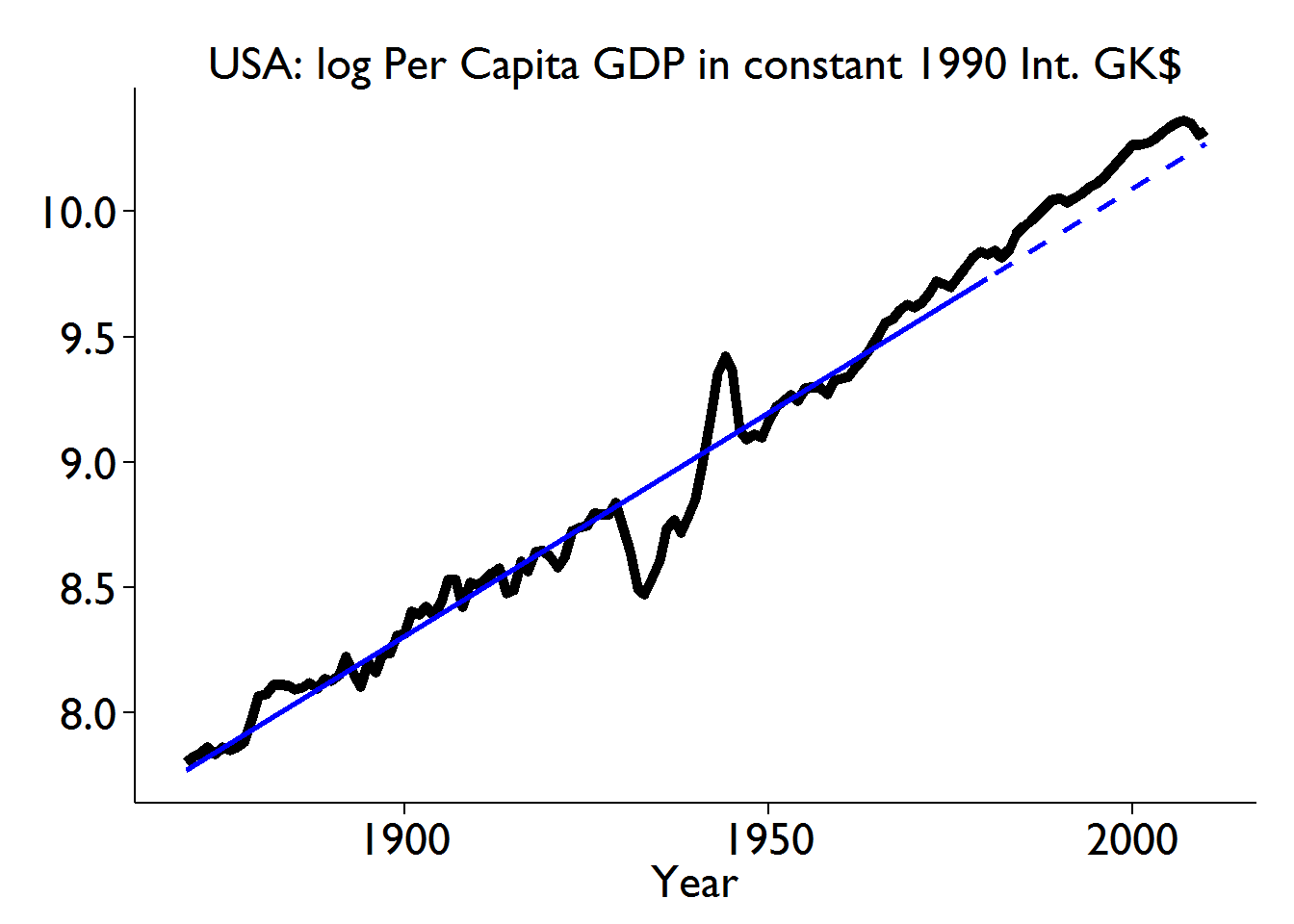``````##
## > thisTitle <- paste0(theEconomy, ": Per Capita GDP in constant 1990 Int. GK\$")
##
## > expTrendFitted <- function(x) {
## +     ifelse(x >= theBegFit & x <= theEndFit, exp(coef(olsFIT) +
## +         (x * coef(olsFIT))), NA)
## + }
##
## > expTrendExtrap <- function(x) {
## +     ifelse(x >= theEndFit + 1 & x <= theEndSmp, exp(coef(olsFIT) +
## +         (x * coef(olsFIT))), NA)
## + }
##
## > ggplot(data = this.DF, aes(x = Year, y = perCapitaGDP)) +
## +     geom_line(size = 2) + stat_function(data = this.DF, fun = expTrendFitted,
## +     li .... [TRUNCATED]``````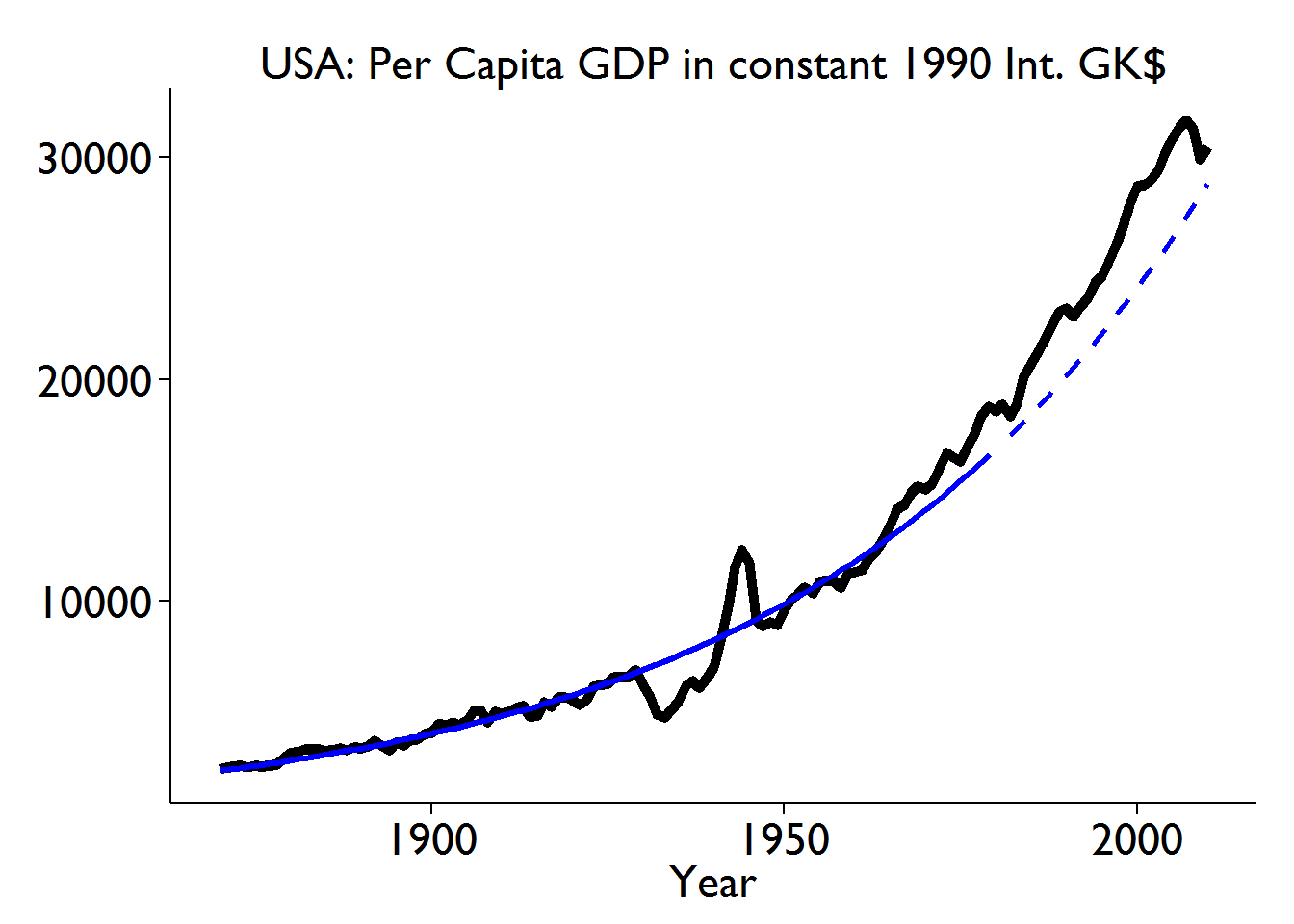``````##
## > rm(olsFIT, expTrendFitted, expTrendExtrap)``````
``rm(theBegFit, theEndFit, theEndSmp, theEconomy)``

where presented are both the fitted linear trend for the log of US per capita GDP, and the resulting exponential trend for the original series. The solid line is the fitted trend; the dashed line the extrapolation.

Remarkably, a smooth exponential trend, fitted from 1870 through as early as 1980, gives a reasonable description on the out-of-sample post-1980 behaviour of US per capita GDP.

Do the same for China but now beginning in 1950 as it’s from then that the Maddison Project data provide a usefully uninterrupted sequence:

``````theBegFit  <- 1950
theEndFit  <- 1980
theEndSmp  <- 2010
theEconomy <- "China"

source(file="./eyetrend.R", local=TRUE, echo=TRUE)``````
``````##
## > olsFIT <- lm(logPerCapGDP ~ Year, data = MaddP.DF[(MaddP.DF\$Economy ==
## +     theEconomy) & (MaddP.DF\$Year >= theBegFit) & (MaddP.DF\$Year <=
## +      .... [TRUNCATED]
##
## > thisTitle <- paste0(theEconomy, ": log Per Capita GDP in constant 1990 Int. GK\$")
##
## > this.DF <- MaddP.DF[(MaddP.DF\$Economy == theEconomy) &
## +     (MaddP.DF\$Year >= theBegFit) & (MaddP.DF\$Year <= theEndSmp),
## +     c("Year", "perCapi ..." ... [TRUNCATED]
##
## > ggplot(data = this.DF, aes(x = Year, y = logPerCapGDP)) +
## +     geom_line(size = 2) + geom_segment(data = this.DF, aes(x = theBegFit,
## +     xend = .... [TRUNCATED]``````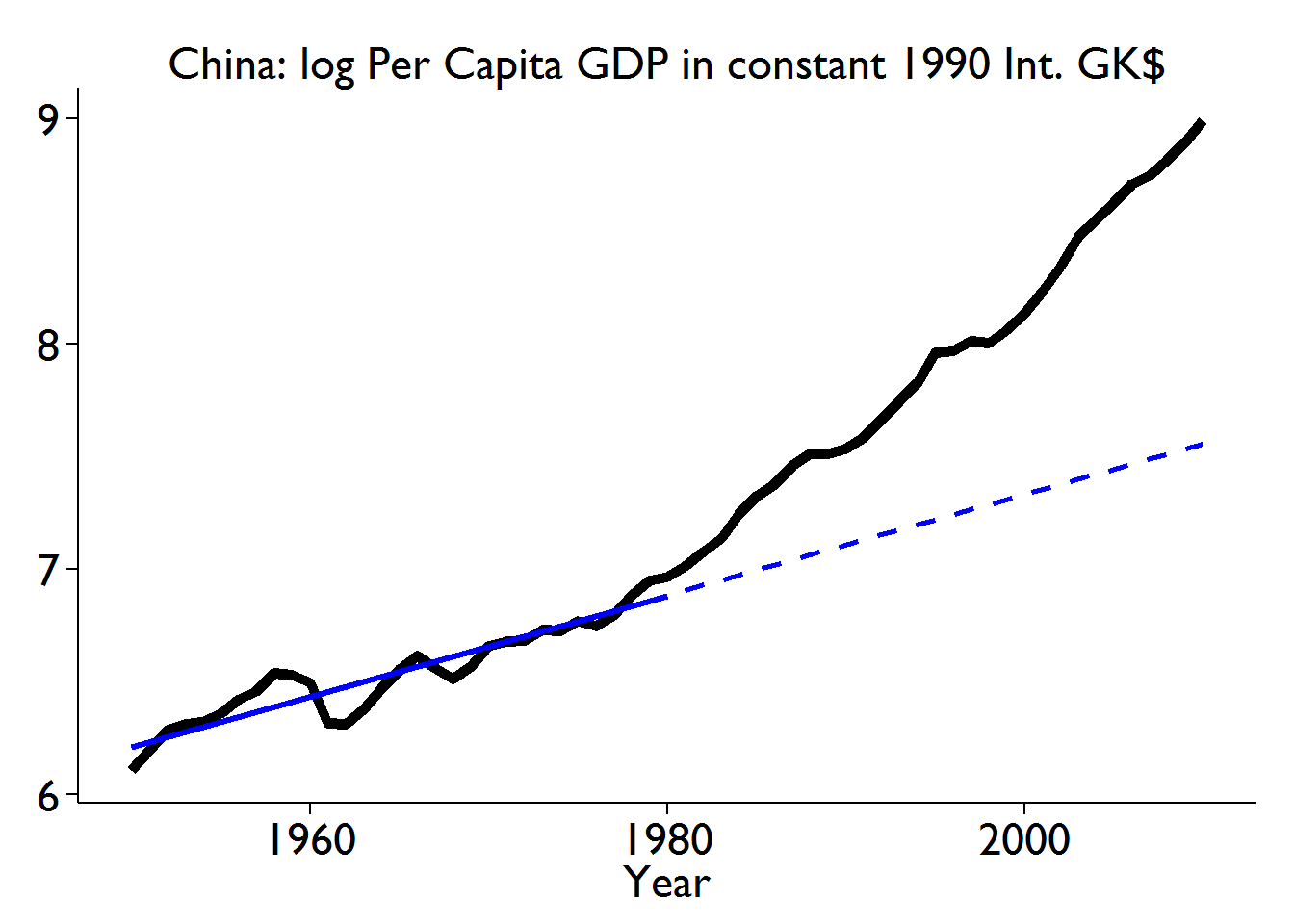``````##
## > thisTitle <- paste0(theEconomy, ": Per Capita GDP in constant 1990 Int. GK\$")
##
## > expTrendFitted <- function(x) {
## +     ifelse(x >= theBegFit & x <= theEndFit, exp(coef(olsFIT) +
## +         (x * coef(olsFIT))), NA)
## + }
##
## > expTrendExtrap <- function(x) {
## +     ifelse(x >= theEndFit + 1 & x <= theEndSmp, exp(coef(olsFIT) +
## +         (x * coef(olsFIT))), NA)
## + }
##
## > ggplot(data = this.DF, aes(x = Year, y = perCapitaGDP)) +
## +     geom_line(size = 2) + stat_function(data = this.DF, fun = expTrendFitted,
## +     li .... [TRUNCATED]``````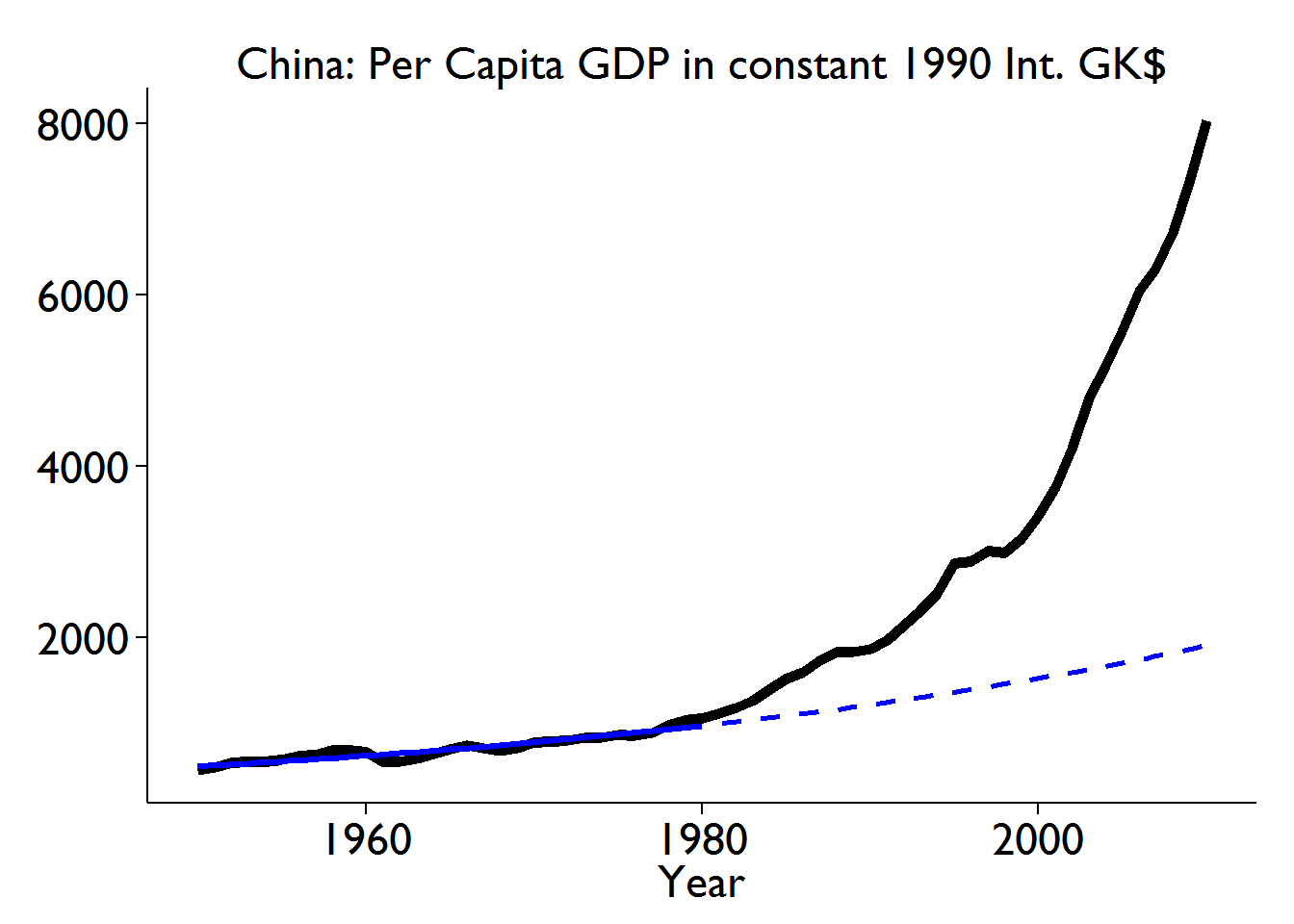``````##
## > rm(olsFIT, expTrendFitted, expTrendExtrap)``````
``rm(theEconomy, theEndSmp, theEndFit, theBegFit)``

In stark contrast to the US, China’s per capita GDP follows post-1980 a completely different trajectory from its pre-1980 history. This, of course, is no surprise to anyone even vaguely aware of global economic developments. The value of the calculation is to quantify how large the change is that has occurred: if anyone thought growth trends were slow and difficult to change, China provides a striking and positive counter-example.

Finally, for comparison, let’s do this for the UK:

``````theBegFit  <- 1950
theEndFit  <- 1980
theEndSmp  <- 2010
theEconomy <- "England/GB/UK"

source(file="./eyetrend.R", local=TRUE, echo=TRUE)``````
``````##
## > olsFIT <- lm(logPerCapGDP ~ Year, data = MaddP.DF[(MaddP.DF\$Economy ==
## +     theEconomy) & (MaddP.DF\$Year >= theBegFit) & (MaddP.DF\$Year <=
## +      .... [TRUNCATED]
##
## > thisTitle <- paste0(theEconomy, ": log Per Capita GDP in constant 1990 Int. GK\$")
##
## > this.DF <- MaddP.DF[(MaddP.DF\$Economy == theEconomy) &
## +     (MaddP.DF\$Year >= theBegFit) & (MaddP.DF\$Year <= theEndSmp),
## +     c("Year", "perCapi ..." ... [TRUNCATED]
##
## > ggplot(data = this.DF, aes(x = Year, y = logPerCapGDP)) +
## +     geom_line(size = 2) + geom_segment(data = this.DF, aes(x = theBegFit,
## +     xend = .... [TRUNCATED]``````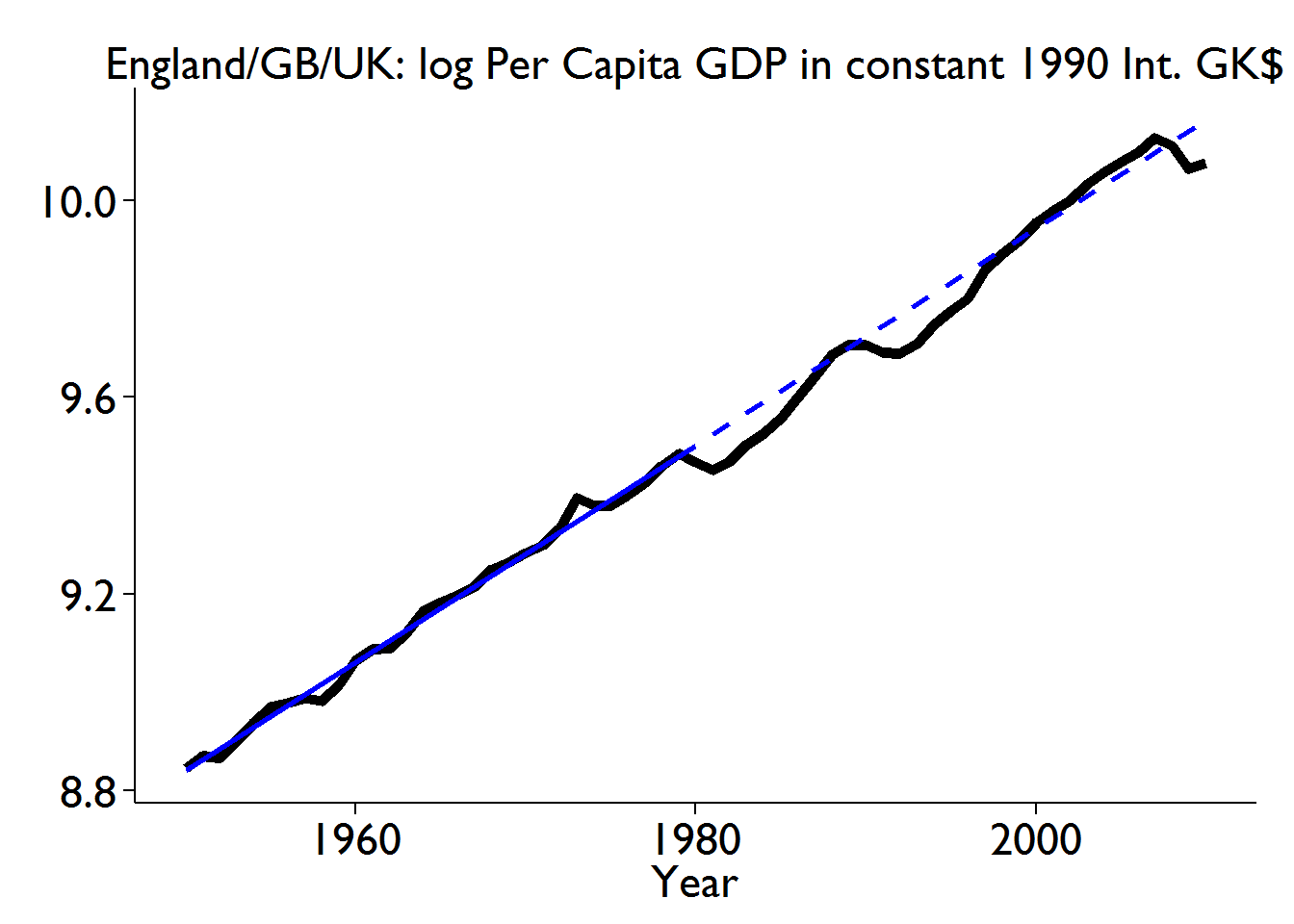``````##
## > thisTitle <- paste0(theEconomy, ": Per Capita GDP in constant 1990 Int. GK\$")
##
## > expTrendFitted <- function(x) {
## +     ifelse(x >= theBegFit & x <= theEndFit, exp(coef(olsFIT) +
## +         (x * coef(olsFIT))), NA)
## + }
##
## > expTrendExtrap <- function(x) {
## +     ifelse(x >= theEndFit + 1 & x <= theEndSmp, exp(coef(olsFIT) +
## +         (x * coef(olsFIT))), NA)
## + }
##
## > ggplot(data = this.DF, aes(x = Year, y = perCapitaGDP)) +
## +     geom_line(size = 2) + stat_function(data = this.DF, fun = expTrendFitted,
## +     li .... [TRUNCATED]``````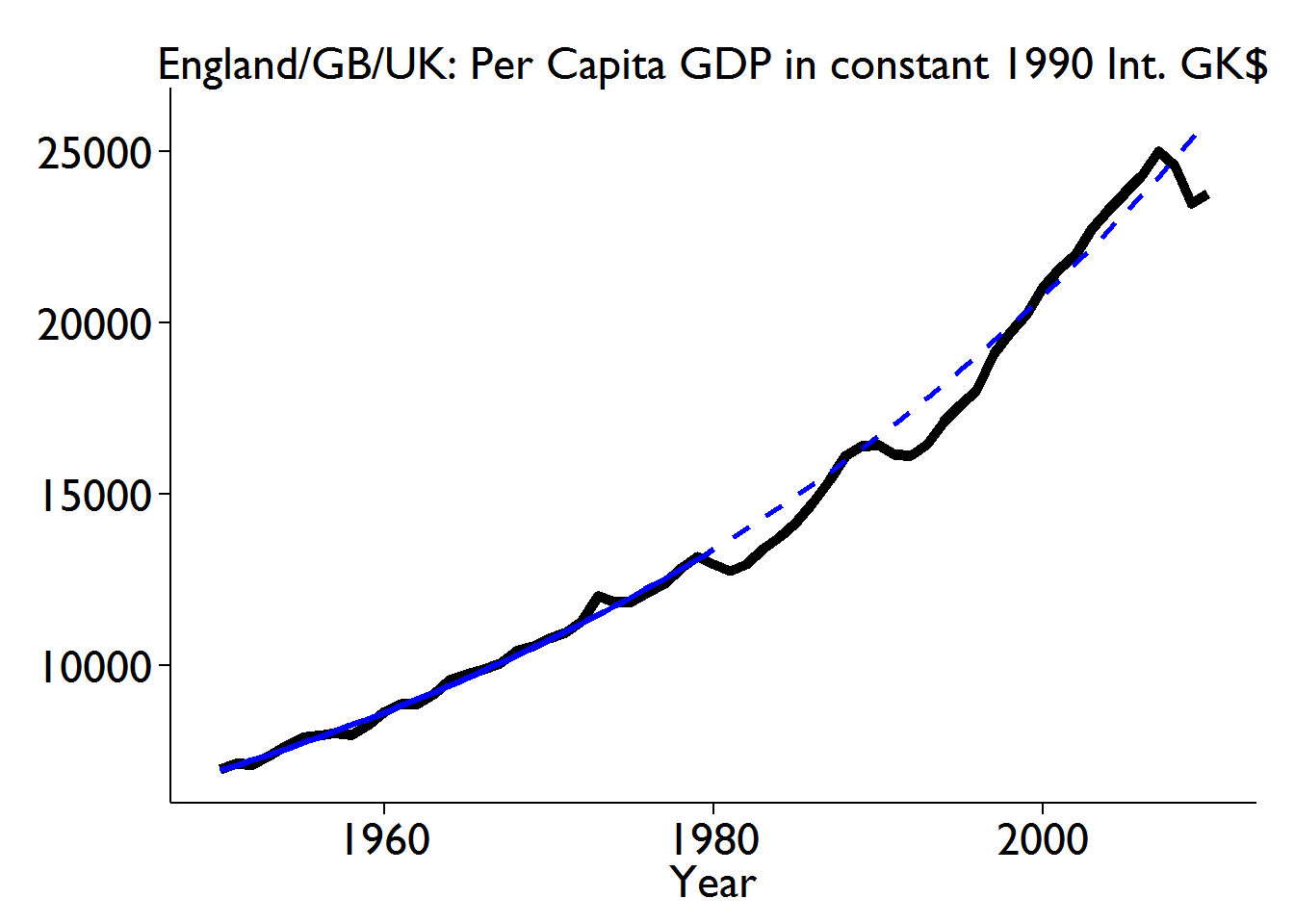``````##
## > rm(olsFIT, expTrendFitted, expTrendExtrap)``````
``rm(theEconomy, theEndSmp, theEndFit, theBegFit)``

Get a final sense of the difference here by putting all these on the same graph.

``````theBegSmpl   <- 1950
theEndSmpl   <- 2010
theEconomies <- c("USA", "England/GB/UK", "China")
theSeries    <- "logPerCapGDP"
thisTitle    <- "log Per Capita GDP in constant 1990 Int. GK\$"

source(file="./multplot.R", local=TRUE, echo=TRUE)``````
``````##
## > getSeries <- c("Year", "Economy", theSeries)
##
## > theAES <- aes_string(x = "Year", y = theSeries, group = "Economy",
## +     colour = "Economy")
##
## > this.DF <- MaddP.DF[(MaddP.DF\$Economy %in% theEconomies) &
## +     (MaddP.DF\$Year >= theBegSmpl) & (MaddP.DF\$Year <= theEndSmpl),
## +     getSeries]
##
## > ggplot(data = this.DF, theAES) + geom_line(size = 2) +
## +     myTStheme + ggtitle(thisTitle)``````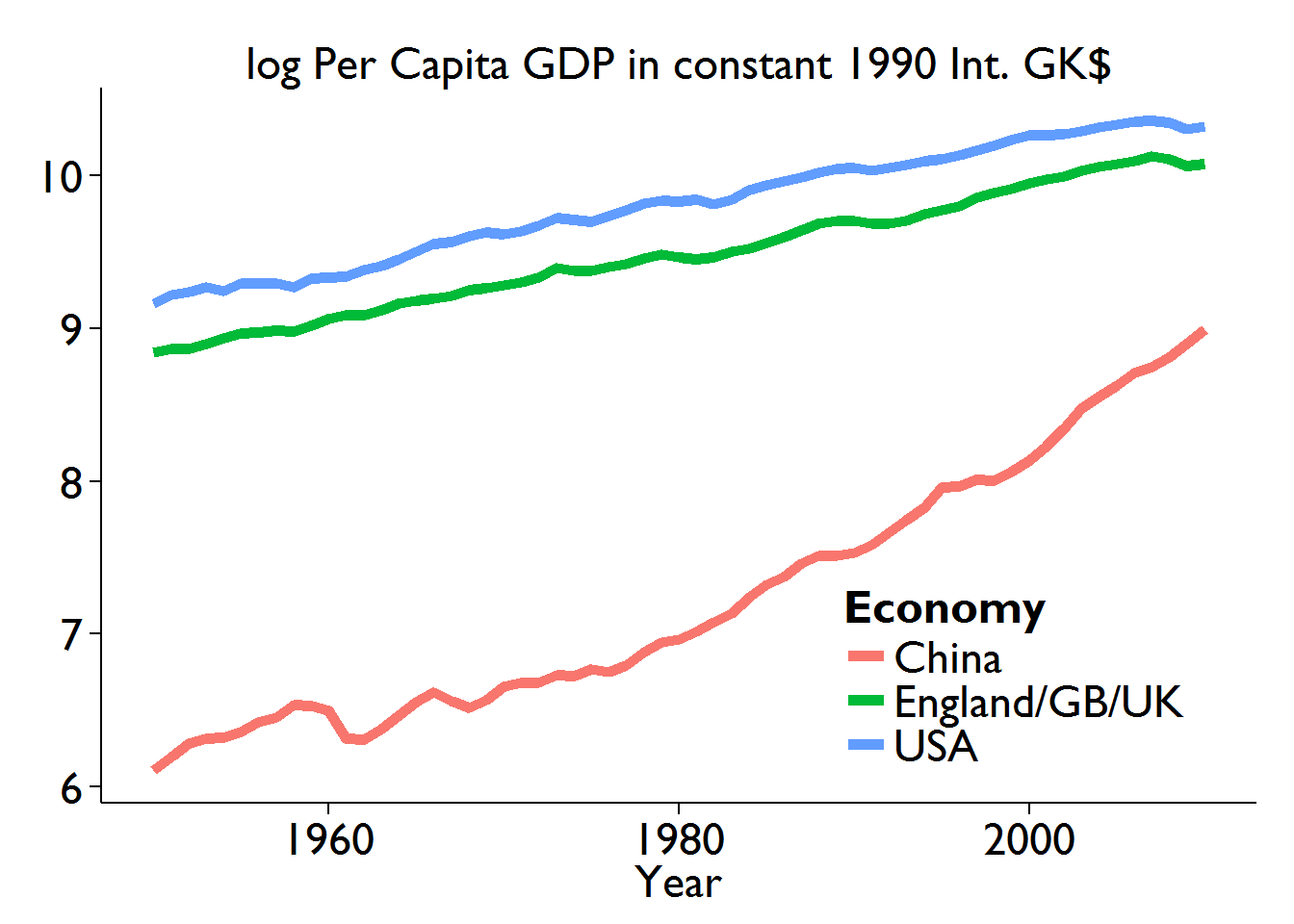``````##
## > rm(this.DF, theAES, getSeries)``````
``rm(thisTitle, theSeries, theEconomies, theEndSmpl, theBegSmpl)``

A more useful perspective on the size of these cross-country differences come from the levels of the series themselves, not their logs.

``````myTStheme <- theme_classic() +
theme(
plot.title=element_text(size=rel(1.5)),
legend.title=element_text(size=rel(1.5)),
legend.text=element_text(size=rel(1.5)),
legend.position=c(0.45,0.6), legend.justification=c(1,0),
axis.text=element_text(size=rel(1.5)),
axis.title=element_text(size=rel(1.5)),
axis.title.y=element_blank()
)``````
``````theBegSmpl   <- 1950
theEndSmpl   <- 2010
theEconomies <- c("USA", "England/GB/UK", "China")
theSeries    <- "perCapitaGDP"
thisTitle    <- "Per Capita GDP in constant 1990 Int. GK\$"

source(file="./multplot.R", local=TRUE, echo=TRUE)``````
``````##
## > getSeries <- c("Year", "Economy", theSeries)
##
## > theAES <- aes_string(x = "Year", y = theSeries, group = "Economy",
## +     colour = "Economy")
##
## > this.DF <- MaddP.DF[(MaddP.DF\$Economy %in% theEconomies) &
## +     (MaddP.DF\$Year >= theBegSmpl) & (MaddP.DF\$Year <= theEndSmpl),
## +     getSeries]
##
## > ggplot(data = this.DF, theAES) + geom_line(size = 2) +
## +     myTStheme + ggtitle(thisTitle)``````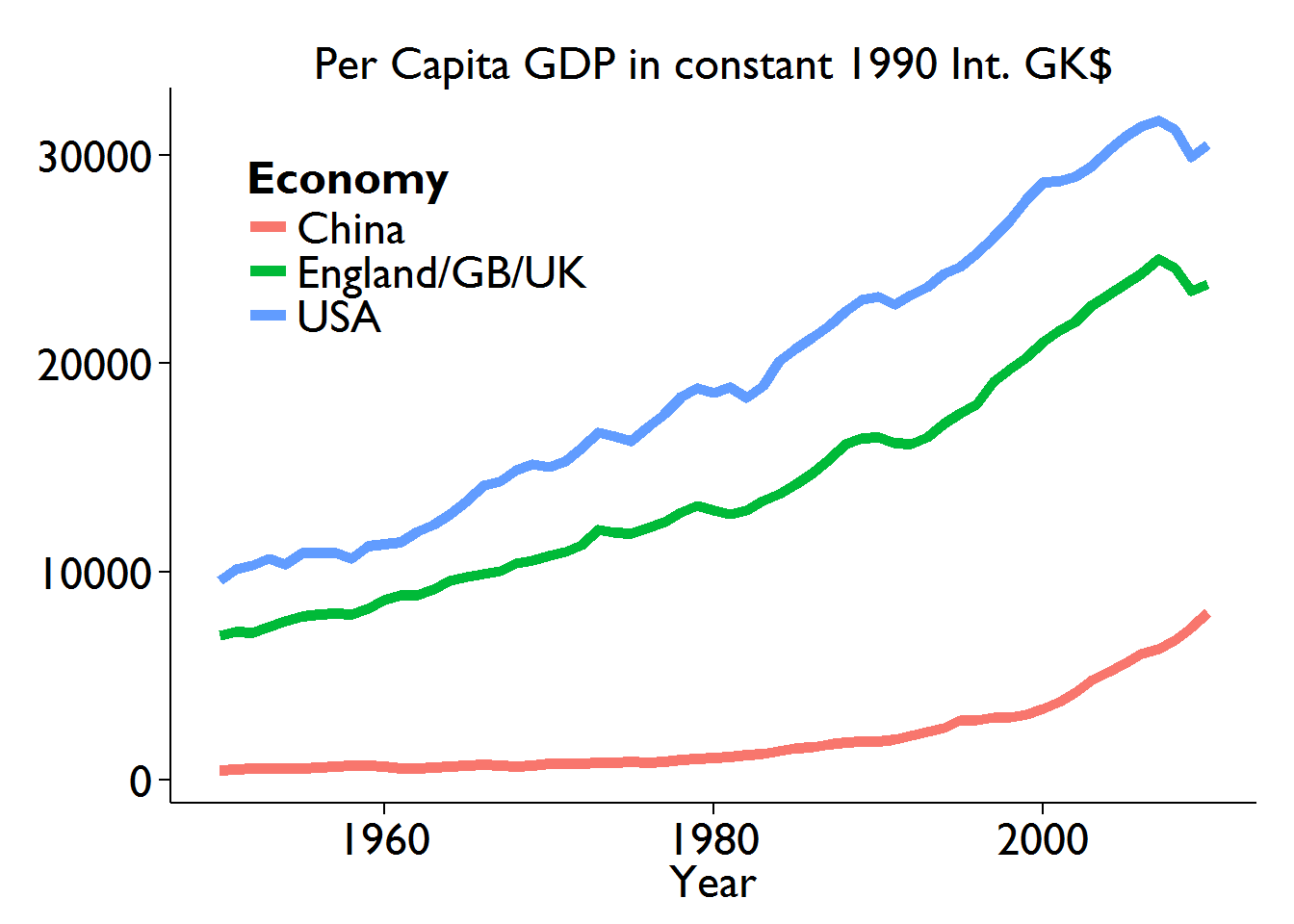``````##
## > rm(this.DF, theAES, getSeries)``````
``rm(thisTitle, theSeries, theEconomies, theEndSmpl, theBegSmpl)``

Remember, however, that this is for per capita GDP and obviously therefore does not take into account the sizes of the different populations.

APPENDIX

The is is the code chunk for plotting multiple series neatly:

``read_chunk("./multplot.R")``
``````getSeries <- c("Year", "Economy", theSeries)
theAES    <- aes_string(x="Year", y=theSeries, group="Economy",
colour="Economy")
this.DF   <-
(MaddP.DF\$Year >= theBegSmpl) & (MaddP.DF\$Year <= theEndSmpl),
getSeries]

ggplot(data=this.DF, theAES) + geom_line(size=2) +
myTStheme + ggtitle(thisTitle)

rm(this.DF, theAES, getSeries)``````

This is the code chunk that implements the eyeballing-trend operation.

``read_chunk("./eyetrend.R")``
``````olsFIT <- lm(logPerCapGDP ~ Year,
(MaddP.DF\$Year >= theBegFit) & (MaddP.DF\$Year <= theEndFit), ])

thisTitle <- paste0(theEconomy,
": log Per Capita GDP in constant 1990 Int. GK\$")

this.DF <-
(MaddP.DF\$Year >= theBegFit) & (MaddP.DF\$Year <= theEndSmp),
c("Year", "perCapitaGDP", "logPerCapGDP")]

ggplot(data=this.DF, aes(x=Year, y=logPerCapGDP)) + geom_line(size=2) +
geom_segment(data=this.DF, aes(x=theBegFit, xend=theEndFit,
y=coef(olsFIT)+coef(olsFIT)*theBegFit,
yend=coef(olsFIT)+coef(olsFIT)*theEndFit),
linetype=1, colour="blue", size=1.1) +
geom_segment(data=this.DF, aes(x=theEndFit+1, xend=theEndSmp,
y=coef(olsFIT)+coef(olsFIT)*(theEndFit+1),
yend=coef(olsFIT)+coef(olsFIT)*theEndSmp),
linetype=2, colour="blue", size=1.05) +
myTStheme + ggtitle(thisTitle)

#
thisTitle      <- paste0(theEconomy,
": Per Capita GDP in constant 1990 Int. GK\$")

expTrendFitted <- function(x) {ifelse (x>=theBegFit & x<=theEndFit,
exp(coef(olsFIT) + (x * coef(olsFIT))), NA)
}
expTrendExtrap <- function(x) {ifelse (x>=theEndFit+1 & x<=theEndSmp,
exp(coef(olsFIT) + (x * coef(olsFIT))), NA)
}
ggplot(data=this.DF, aes(x=Year, y=perCapitaGDP)) + geom_line(size=2) +
stat_function(data=this.DF, fun=expTrendFitted, linetype=1,
colour="blue", size=1.1) +
stat_function(data=this.DF, fun=expTrendExtrap, linetype=2,
colour="blue", size=1.05) +
myTStheme + ggtitle(thisTitle)

rm(olsFIT, expTrendFitted, expTrendExtrap)``````